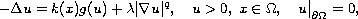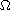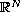Electron. J. Diff. Eqns., Vol. 2006(2006), No. 93, pp. 1-8.

### Asymptotic behaviour of the solution for the singular Lane-Emden-Fowler equation with nonlinear convection terms Zhijun Zhang

Abstract:
We show the exact asymptotic behaviour near the boundary for the classical solution to the Dirichler problemwhereis a bounded domain with smooth boundary in. We use the Karamata regular varying theory, a perturbed argument, and constructing comparison functions.

Submitted December 23, 2005. Published August 18, 2006.
Math Subject Classifications: 35J65, 35B05, 35O75, 35R05.
Key Words: Semilinear elliptic equations; Dirichlet problem; singularity; nonlinear convection terms; Karamata regular variation theory; unique solution; exact asymptotic behaviour.

Show me the PDF file (232K), TEX file, and other files for this article.Zhijun Zhang Department of Mathematics and Informational Science Yantai University, Yantai, Shandong, 264005, China email: zhangzj@ytu.edu.cn